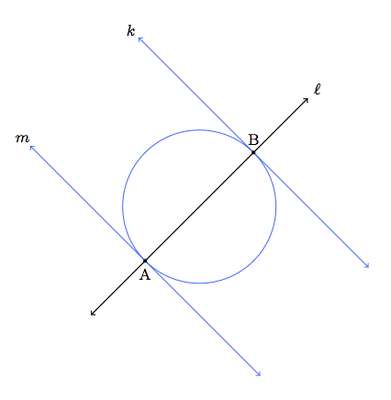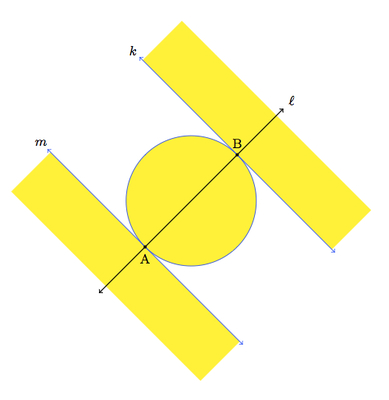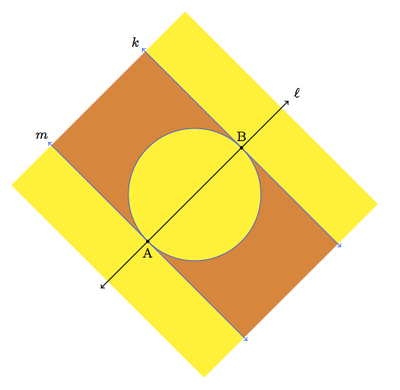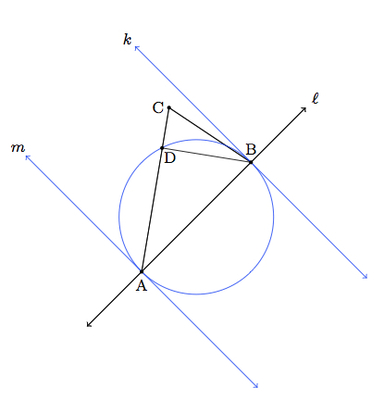# Classifying Triangles

Alignments to Content Standards: G-CO.C.10

Choose two distinct points $A$ and $B$ in the plane.

1. For which points $C$ is $\triangle ABC$ a right triangle?
2. For which points $C$ is $\triangle ABC$ an obtuse triangle (that is, a triangle with one obtuse angle)?
3. For which points $C$ is $\triangle ABC$ an acute triangle (that is, a triangle with three acute angles)?

## IM Commentary

The goal of this task is to help students synthesize their knowledge of triangles. They will need to know that a triangle inscribed in a circle, with a diameter of the circle as one side, is a right triangle. They may also need some help getting started with the task because the question is about triangles and only two of the vertices have been given. The teacher should encourage students to experiment by choosing some different points for the third vertex. After all students have selected a few points and decided whether or not those triangles are acute, obtuse, or right, they should share their results with one another. After this experimental stage, students can begin to think about which points in the plane correspond to which of the three different types of triangle.

For the triangles which are acute and obtuse, a wide range of arguments should be expected with the most likely being an informal argument based on ''appearances.'' This type of argument is important and the teacher will need to decide, based on the knowledge of the students, if a more formal argument is desired. Formal arguments have been included in the solution below.

This task embodies MP7, ''Look For and Make Use of Structure'' since the different triangles divide the plane into very simple regions. This is to be expected since, as we move the vertex $C$ of the triangle, in order to pass from an acute triangle to an obtuse triangle, one of the angles must at some moment be a right angle. Therefore the key in this problem is to identify the right triangles and then this gives the regions of the plane for the different possibilities. If geometry software is available to help in this exploration, it would be very useful, both for helping the students to readily produce many different triangles and also for helping them verify in some cases that the triangles are acute, right, or obtuse. In this case, the task can also serve to illustrate MP5, Use Appropriate Tools Strategically. This standard is also appropriate if students use straightedge and compass to construct the blue circle and lines showing the right triangles the solution.

## Solution

Here are our points $A$ and $B$:1. First of all, the point $C$ can not be collinear with $A$ and $B$ if $ABC$ forms a triangle. So we need to look at all points except for those on $\overleftrightarrow{AB}$.

For the right triangles with $\overline{AB}$ as one side, there are three possibilities: the right angle could be at vertex $A$, $B$, or $C$. If the right angle is at vertex $A$ this means that $C$ lies on the line perpendicular to $\overleftrightarrow{AB}$ at $A$. If the right angle is at vertex $B$ then $C$ lies on the line perpendicular to $\overleftrightarrow{AB}$ at $B$. Finally, if the right angle is at $C$, this means that the $C$ lies on the circle with diameter $\overline{AB}$. This is pictured below with the points $C$ making a right triangle $ABC$ in blue:2. Like in part (a), there are three possibilities as the obtuse angle can be at vertex $A$, at vertex $B$, or at vertex $C$. When the obtuse angle is at vertex $A$ this means that $C$ is to the left and below the perpendicular line to $\overleftrightarrow{AB}$ at $A$. Similarly when the obtuse angle is at vertex $B$ the vertex $C$ must be to the right and above the perpendicular line to $\overleftrightarrow{AB}$ at $B$. Finally, when the obtuse angle is at $C$ this means that vertex $C$ is on the interior of the circle with diameter $\overline{AB}$ (though not on $\overline{AB}$). This is pictured below with all of the possible locations of $C$ making an obtuse triangle with $A$ and $B$ shaded in yellow:For a point $C$, for example, in the upper unbounded yellow region $m(\angle ABC) > 90$ because angle $ABC$ contains, in its interior, one of the right angles made by $k$ with $\ell = \overleftrightarrow{AB}$. A similar argument applies to the lower unbounded yellow region. For the interior yellow region, we provide a picture which will help explain why the angle at $C$ is greater than 90$^\circ$.We know from part (a) that $m(\angle ADB) = 90$. We have $$m(\angle BAD) + m(\angle DBA) + m(\angle ADB) = 180.$$ This gives $$m(\angle BAD) + m(\angle DBA) = 90.$$ Angles $BAC$ and $BAD$ are the same angle and $m(\angle DBA) \gt m(\angle CBA)$. Thus $$m(\angle BAC) + m(\angle CBA) \lt 90.$$ Since $$m(\angle BAC) + m(\angle CBA) + m(\angle ACB) = 180$$ we conclude as desired that $m(\angle ACB) \gt 90$ and hence $\triangle ABC$ is obtuse.

3. Everything that is left after filling in the right and obtuse triangles must be acute! This means that the regions shaded orange give acute triangles:We can show that these triangles are acute in a way very similar to the argument in part (b). Here is a picture of this situation:We know from part (a) that $m(\angle ADB) = 90$. We have $$m(\angle BAD) + m(\angle DBA) + m(\angle ADB) = 180.$$ This gives $$m(\angle BAD) + m(\angle DBA) = 90.$$ Angles $BAC$ and $BAD$ are the same angle and $m(\angle DBA) \lt m(\angle CBA)$. Thus $$m(\angle BAC) + m(\angle CBA) \gt 90.$$ Since $$m(\angle BAC) + m(\angle CBA) + m(\angle ACB) = 180$$ we conclude as desired that $m(\angle ACB) \lt 90$ and hence $\triangle ABC$ is acute.